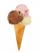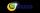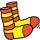Tournament

Determine how many ways can be chosen two representatives from 34 students to school tournament.

Result

n =  561

Solution:Leave us a comment of this math problem and its solution (i.e. if it is still somewhat unclear...):Be the first to comment!To solve this verbal math problem are needed these knowledge from mathematics:

Would you like to compute count of combinations?

Next similar math problems:

1. ExaminationThe class is 21 students. How many ways can choose two to examination?
2. Fish tankA fish tank at a pet store has 8 zebra fish. In how many different ways can George choose 2 zebra fish to buy?
3. ConfectioneryThe village markets have 5 kinds of sweets, one weighs 31 grams. How many different ways a customer can buy 1.519 kg sweets.
4. TeamsHow many ways can divide 16 players into two teams of 8 member?
5. Ice creamAnnie likes much ice cream. In the shop are six kinds of ice cream. In how many ways she can buy ice cream to three scoop if each have a different flavor mound and the order of scoops doesn't matter?
6. TrinityHow many different triads can be selected from the group 43 students?
7. MATESIn MATES (Small Television tipping) from 35 randomly numbers drawn 5 winning numbers. How many possible combinations there is?
8. Volleyball8 girls wants to play volleyball against boys. On the field at one time can be six players per team. How many initial teams of this girls may trainer to choose?
9. ChordsHow many 4-tones chords (chord = at the same time sounding different tones) is possible to play within 7 tones?
10. Class pairsIn a class of 34 students, including 14 boys and 20 girls. How many couples (heterosexual, boy-girl) we can create? By what formula?
11. BlocksThere are 9 interactive basic building blocks of an organization. How many two-blocks combinations are there?
12. Theorem proveWe want to prove the sentence: If the natural number n is divisible by six, then n is divisible by three. From what assumption we started?
13. Calculation of CNCalculate: ?
14. Cancel fractionsCompress the expression of factorial: (n+6)!/(n+4)!-n!/(n-2)!
15. Functions f,gFind g(1) if g(x) = 3x - x2 Find f(5) if f(x) = x + 1/2
16. PowersExpress the expression ? as the n-th power of the base 10.
17. First manWhat is the likelihood of a random event where are five men and seven women first will leave the man?